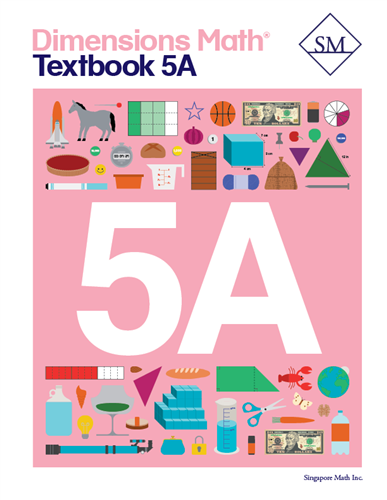# Dimensions Math Textbook 5A

\$ 12.00

Dimensions Math® Textbook 5A

Chapter 1: Numbers to One Million

Lesson 1: Numbers to 100,000
Lesson 2: Numbers to 1,000,000
Lesson 3: Number Patterns
Lesson 4: Comparing and Ordering Numbers
Lesson 5: Rounding 5-Digit Numbers
Lesson 6: Rounding 6-Digit Numbers
Lesson 7: Calculations and Place Value
Lesson 8: Practice

Lesson 2: Subtraction
Lesson 3: Other Ways to Add and Subtract — Part 1
Lesson 4: Other Ways to Add and Subtract — Part 2
Lesson 5: Word Problems
Lesson 6: Practice

Chapter 3: Multiples and Factors

Lesson 1: Multiples
Lesson 2: Common Multiples
Lesson 3: Factors
Lesson 4: Prime Numbers and Composite Numbers
Lesson 5: Common Factors
Lesson 6: Practice

Chapter 4: Multiplication

Lesson 1: Mental Math for Multiplication
Lesson 2: Multiplying by a 1-Digit Number — Part 1
Lesson 3: Multiplying by a 1-Digit Number — Part 2
Lesson 4: Practice A
Lesson 5: Multiplying by a Multiple of 10
Lesson 6: Multiplying by a 2-Digit Number — Part 1
Lesson 7: Multiplying by a 2-Digit Number — Part 2
Lesson 8: Practice B

Chapter 5: Division

Lesson 1: Mental Math for Division
Lesson 2: Estimation and Division
Lesson 3: Dividing 4-Digit Numbers
Lesson 4: Practice A
Lesson 5: Word Problems
Lesson 6: Challenging Word Problems
Lesson 7: Practice B
Review 1

Chapter 6: Fractions

Lesson 1: Equivalent Fractions
Lesson 2: Comparing and Ordering Fractions
Lesson 3: Improper Fractions and Mixed Numbers
Lesson 4: Practice A
Lesson 5: Expressing an Improper Fraction as a Mixed Number
Lesson 6: Expressing a Mixed Number as an Improper Fraction
Lesson 7: Fractions and Division
Lesson 8: Practice B

Chapter 7: Adding and Subtracting Fractions

Lesson 1: Adding and Subtracting Fractions — Part 1
Lesson 2: Adding and Subtracting Fractions — Part 2
Lesson 3: Adding a Mixed Number and a Fraction
Lesson 5: Subtracting a Fraction from a Mixed Number
Lesson 6: Subtracting Mixed Numbers
Lesson 7: Practice

Chapter 8: Multiplying a Fraction and a Whole Number

Lesson 1: Multiplying a Unit Fraction by a Whole Number
Lesson 2: Multiplying a Fraction by a Whole Number — Part 1
Lesson 3: Multiplying a Fraction by a Whole Number — Part 2
Lesson 4: Fraction of a Set
Lesson 5: Multiplying a Whole Number by a Fraction — Part 1
Lesson 6: Multiplying a Whole Number by a Fraction — Part 2
Lesson 7: Word Problems — Part 1
Lesson 8: Word Problems — Part 2

Lesson 9: Practice

Chapter 9: Line Graphs and Line Plots

Lesson 1: Line Graphs
Lesson 2: Drawing Line Graphs
Lesson 3: Line Plots
Lesson 4: Practice
Review 2

Dimensions Math® PreK-5 series features the progression, rigor, and pacing that define Singapore math. Throughout the series, five characters offer students suggestions on how to think about problems. They remind students of strategies they’ve learned and point out important information that encourages them to come up with their own solutions.

Textbook lessons begin with a task that allows students to apply their previous knowledge and learn through discussion. Once students have mastered a concept with the use of concrete and pictorial aids, they are ready to take on more abstract mathematical problem sets. They reach fluency by collecting various strategies along the way and applying them to new problems. Word problems give students a sense of math in real-world contexts.

Workbooks offer independent practice that follows a careful progression of exercise variation. Each textbook lesson includes a corresponding workbook exercise that starts with pictorial representation and progresses to more challenging abstract problems. Workbooks for PreK-2 are perforated.

Teacher’s Guides include lesson plans, mathematical background, games, helpful suggestions, and comprehensive resources for daily lessons. Lessons are laid out clearly and activities are designed for the whole class, small groups, and extension.

Textbooks and Workbooks do not include answer keys. Answers are in Teacher's Guides.
Textbook:
ISBN  9781947226128